Next: Worked example 2.3: The Up: Motion in 1 dimension Previous: Worked example 2.1: Velocity-time

## Worked example 2.2: Speed trap

Question: In a speed trap, two pressure-activated strips are placed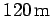apart on a highway on which the speed limit is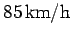. A driver going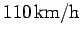notices a police car just as he/she activates the first strip, and immediately slows down. What deceleration is needed so that the car's average speed is within the speed limit when the car crosses the second strip?

Answer: Let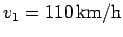be the speed of the car at the first strip. Let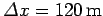be the distance between the two strips, and let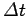be the time taken by the car to travel from one strip to the other. The average velocity of the car is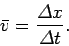We need this velocity to be. Hence, we require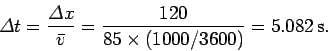Here, we have changed units from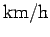to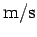. Now, assuming that the accelerationof the car is uniform, we have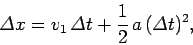which can be rearranged to give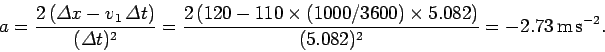Hence, the required deceleration is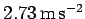.Next: Worked example 2.3: The Up: Motion in 1 dimension Previous: Worked example 2.1: Velocity-time
Richard Fitzpatrick 2006-02-02